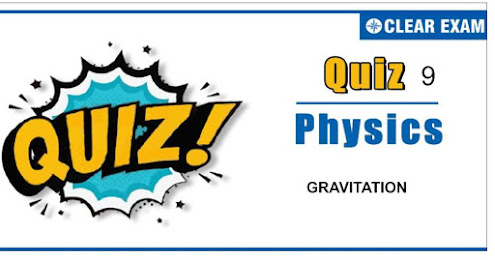## GRAVITATION QUIZ-9

JEE Advanced Physics Syllabus can be referred by the IIT aspirants to get a detailed list of all topics that are important in cracking the entrance examination. JEE Advanced syllabus for Physics has been designed in such a way that it offers very practical and application-based learning to further make it easier for students to understand every concept or topic by correlating it with day-to-day experiences. In comparison to the other two subjects, the syllabus of JEE Advanced for physics is developed in such a way so as to test the deep understanding and application of concepts.

Gravitation is an easy to understand and a highly scoring topic. It might have a 1% weightage in the entire JEE Physics portion but do not neglect. You can practice these questions on regular basis to know what are the important points to remember at the moment when gravitation question comes.

Q1.Two concentric shells have masses M and m and their radii are R and r, respectively, where R>r. What is the gravitational potential at their common centre?

•  -GM/R
•  -GM/r
•  -G[M/R - m/r]
•  -G[M/R + m/r]
Q2.The following figure shows two shells of masses m1 and m2. The shells are concentric. At which point, a particle of mass m shall experience zero force?
•  A
•  B
•  C
•  D
Q3.Two rings having masses M and 2M, respectively, having the same radius are placed coaxially as shown in the figure If the mass distribution on both the rings is non-uniform, then the gravitational potential at point P is

•  -GM/R [1/√2 + 2/√5]
•  -GM[1  +  2/2]/R
•  Zero
•  Cannot be determined from the given information
Q4.The gravitational force exerted by the Sun on the Moon is about twice as great as the gravitational force exerted by the earth on the Moon, but still Moon is not escaping from the gravitational influence of the earth. Mark the option which correctly explains the above system
•  Escape speed is independent of the direction in which it is projected
•  The rotational effect of the earth plays a role in computation of escape speed, however small it may be
•  A body thrown in the eastward direction has less escape speed
•  None of the above
Q5.A satellite of mass m revolves around the earth of radius R at a height x from its surface. If g is the acceleration due to gravity on the surface of the earth, the orbital speed of the satellite is
•  gx
•  gR/(R-x)
•  (gR2)/(R+x)
•  ((gR2)/(R+x))(1/2)
Q6.In problem 83, what is the gravitational field at the centre of the cavities?
•  31GM/1024
•  Gm/1024
•  31GM
•  GM
Q7. If the mass of a planet is 10% less than that of the earth and the radius is 20% greater than that of the earth, the acceleration due to gravity on the planet will be
•  5/8 times that on the surface of the earth
•  3/4 times that on the surface of the earth
•  1/2 times that on the surface of the earth
•  9/10 times that on the surface of the earth
Q8.A satellite is moving with a constant speed v in a circular orbit about the earth. An object of mass m is ejected from the satellite such that it just escapes from the gravitational pull of the earth. At the time of its ejection, the kinetic energy of the object is
•  mv2/2
•  mv2
•  3mv2/2
•  2mv2
Q9.A solid sphere of radius R/2 is cut out of a solid sphere of radius R such that the spherical cavity so formed touches the surface on one side and the center of the sphere on the other side, as shown. The initial mass of the solid sphere was M. If a particle of mass m is placed at a distanace 2.5 R from the center of the cavity, then what is the gravitational attraction on the mass m?
•  GMm/(R2)
•  GMm/(2R2)
•  GMm/(8R2)
•  23GMm/100R2
Q10.The gravitational potential of two homogenous spherical shells A and B of same surface density at their respective centres are in the ratio 3:4. If the two shells coalesce into a single one such that surface charge density remains the same, then the ratio of potential at an internal point of the new shell to shell A is equal to
•  3:2
•  4:3
•  5:3
•  5:6#### Written by: AUTHORNAME

AUTHORDESCRIPTION## Want to know more

Please fill in the details below:

## Latest NEET Articles\$type=three\$c=3\$author=hide\$comment=hide\$rm=hide\$date=hide\$snippet=hide

Name

ltr
item
BEST NEET COACHING CENTER | BEST IIT JEE COACHING INSTITUTE | BEST NEET, IIT JEE COACHING INSTITUTE: Gravitation - Quiz 9
Gravitation - Quiz 9
https://1.bp.blogspot.com/-8ukDxFD1fLs/YOGeD09ZEeI/AAAAAAAAEHo/hDsuCAyFz30Zs2YHjY8caTXVnsOmzyDqQCLcBGAsYHQ/w566-h260/Quiz%2BImage%2B20%2B%25288%2529.jpg
https://1.bp.blogspot.com/-8ukDxFD1fLs/YOGeD09ZEeI/AAAAAAAAEHo/hDsuCAyFz30Zs2YHjY8caTXVnsOmzyDqQCLcBGAsYHQ/s72-w566-c-h260/Quiz%2BImage%2B20%2B%25288%2529.jpg
BEST NEET COACHING CENTER | BEST IIT JEE COACHING INSTITUTE | BEST NEET, IIT JEE COACHING INSTITUTE
https://www.cleariitmedical.com/2021/07/gravitation-quiz-9.html
https://www.cleariitmedical.com/
https://www.cleariitmedical.com/
https://www.cleariitmedical.com/2021/07/gravitation-quiz-9.html
true
7783647550433378923
UTF-8